For example, given a data table with the columns A, B, and C, use the following steps to compose the expression A(B + C). Note that this expression is not the same as A x B + C, which evaluates as (A x B) + C.
 1 Click column A in the Columns list.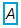2 Click the multiplication button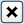in the Formula Editor keypad.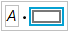3 Enter an open parenthesis.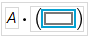4 Click column B in the Columns list.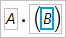5 Click the addition button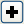in the Formula Editor keypad.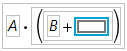6 Click column C in the Columns list.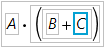For example, using Big Class.jmp, you can predict a child’s height from his age as shown in Figure 6.14. There is a base height of 58.125 inches to which a quantity is added depending on the value of the age variable.
Figure 6.14 The Match Conditional Evaluates Faster Than the If Function
The Match conditional evaluates faster than the If function because the age variable is evaluated only once for each row in the data table. The If condition must evaluate the age variable at each If clause for each row until a clause evaluates as true.

Help created on 7/12/2018